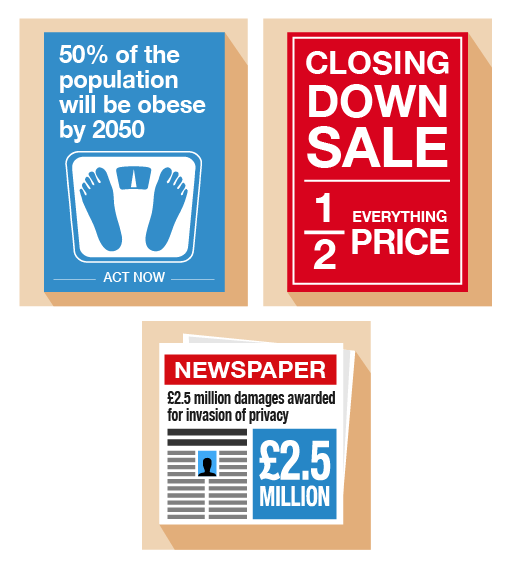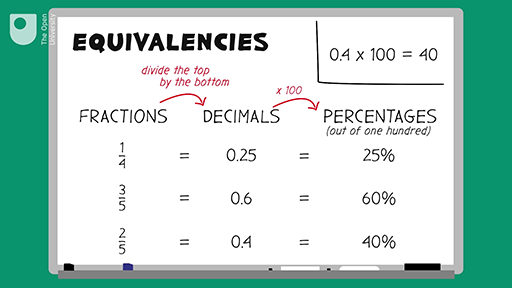Science, Maths & Technology

### Become an OU studentEveryday maths 1 (Wales)

Start this free course now. Just create an account and sign in. Enrol and complete the course for a free statement of participation or digital badge if available.

# 6 Equivalencies between fractions, decimals and percentages

Fractions, decimals and percentages are different ways of saying the same thing. It’s an important skill to learn about the relationships (or ‘equivalencies’) between fractions, decimals and percentages to make sure you are getting the better deal.Figure 31 Looking at equivalencies

Here are some common equivalencies. Try to memorise them – you will come across them a lot in everyday situations:

10% = = 0.1

20% = = 0.2

25% = = 0.25

50% = = 0.5

75% = = 0.75

100% = 1 = 1.0

Look at the following example. If you can identify equivalences, they’ll make it easier to make simple calculations.

## Example: Mine’s a half

What is 50% of £200?

### Method

Since 50% is the same as , so:

50% of £200 = of £200 = £100

Refer to the common equivalencies above (if you need to) to help you with the following activity. Remember to check your answers once you have completed the questions.

## Activity 36: Looking for equivalencies

1. What is 0.75 as a fraction?
2. If you walked 0.25 km each day, what fraction of a kilometer have you walked?
3. House prices have increased by in the last five years. What is this increase as a percentage?
4. A DIY shop is holding a ‘50% off’ sale on kitchens. What is this discount as a fraction?
5. You buy an antique necklace for £3 000. After ten years, its value increases by 20%. What is this increase as a decimal?
6. A headline reads ‘Number of Welsh speakers predicted to rise by 10%’. What is this rise a fraction?
7. What percentage of an hour is 15 minutes?

1. 0.75 as a fraction is .
2. 0.25 is the same as , so you will have walked of a kilometre.
3. is the same as 50%, so the increase is 50%.
4. 50% is the same as , so the discount as a fraction is .
5. 20% is the same as 0.2, so the increase as a decimal is 0.2.
6. 10% is the same as , so according to the headline, the number is predicted to rise by .
7. Think of this as a fraction first: 15 minutes is a quarter () of an hour. is the same as 25%, so 15 minutes is 25% of an hour.

If you find that you are struggling to understand how to convert, please look at the following resource: